• 有个公众号和一些文章用的就是泰坦尼克数据集, 我不知道为什么要用这个，但是我找了很久，还有好多收费的， 我就再次恭喜那一个免费的，让大家的学习门槛低一点。泰坦尼克数据集
• Titanic数据集是非常适合数据科学和机器...数据集为1912年泰坦尼克号沉船事件中一些船员的个人信息以及存活状况。这些历史数据已经非分为训练集和测试集，你可以根据训练集训练出合适的模型并预测测试集中的存活状况。数据集
• 泰坦尼克数据

2018-07-17 23:59:43
titanic数据，包括tran.csv,test.csv,gender_submission.csv,train是编码为1-891共891名乘客的训练数据，test和gender_submission分别为测试数据和需要提交结果的格式以及正确答案。
• 平台下载的原始三个数据train.csv test.csv gender_submission.csv （本来想0积分 分享给大家 无奈最低是1分了）titanic数据
• 泰坦尼克号2201人员的csv数据集。 主要特征有：PassengerId、Pclass、Name 、Sex、Age 、SibSp、 Parch、Ticket 、Fare 、Cabin 、Embarked机器学习
• 正版数据集含代码使用，避免官网下载注册的麻烦。正版数据集含代码使用，避免官网下载注册的麻烦。正版数据集含代码使用，避免官网下载注册的麻烦。
• 泰坦尼克数据集3个csv文件： 1. train.csv， 2. test.csv， 3. gender_submission.csv
• 泰坦尼克号生还情况预测 Kaggle 是一个流行的数据科学竞赛平台，由 Goldbloom 和 Ben Hamner 创建于 2010 年。
• 泰坦尼克数据集，包括train.csv/test.csv/gendermodel.csv
• Abstract The titanic dataset gives the values of four categorical attributes for each of the 2201 people on board the Titanic when it struck an iceberg and sank. The attributes are social class ...
• 泰坦尼克数据集下载 训练集 测试集 导入需要的库 import matplotlib.pyplot as plt %matplotlib inline import numpy as np import pandas as pd 加载数据集 train_file = './data/titanic/train.csv' eval_file = ...
• 提供的训练数据集包含11个特征，分别是：Survived:0代表死亡，1代表存活；Pclass:乘客所持票类，有三种值(1,2,3)；Name:乘客姓名；Sex:乘客性别；Age:乘客年龄(有缺失)；SibSp:乘客兄弟姐妹/配偶的个数(整数值)；...
• 我们拿到的数据通常是不干净的，所谓的不干净，就是数据中有缺失值，有一些异常点等，需要经过一定的处理才能继续做后面的分析或建模，所以拿到数据的第一步是进行数据清洗，本章我们将学习缺失值、重复值、字符串和...

2 第二章：数据清洗及特征处理

我们拿到的数据通常是不干净的，所谓的不干净，就是数据中有缺失值，有一些异常点等，需要经过一定的处理才能继续做后面的分析或建模，所以拿到数据的第一步是进行数据清洗，本章我们将学习缺失值、重复值、字符串和数据转换等操作，将数据清洗成可以分析或建模的亚子。

2.1 缺失值观察与处理

我们拿到的数据经常会有很多缺失值，比如我们可以看到Cabin列存在NaN，那其他列还有没有缺失值，这些缺失值要怎么处理呢

2.1.1 任务一：缺失值观察

(1) 请查看每个特征缺失值个数

查看数据信息

df.info()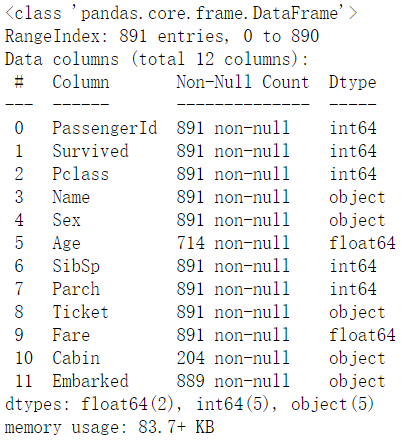查看缺失值个数

df.isnull().sum()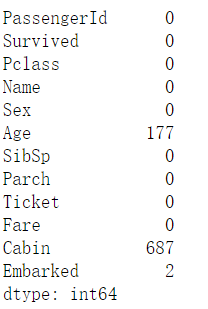(2) 请查看Age， Cabin， Embarked列的数据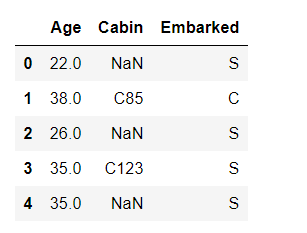2.1.2 任务二：对缺失值进行处理

(1)处理缺失值一般有几种思路

(2) 请尝试对Age列的数据的缺失值进行处理

(3) 请尝试使用不同的方法直接对整张表的缺失值进行处理

#使用年龄均值填补缺失值
df.loc[:,"Age"].fillna(df.loc[:,"Age"].mean(), inplace = True)
df.loc[:,"Age"].isnull().sum()
#删除Cabin列
df.drop("Cabin", axis=1, inplace=True)
#删除Embarked缺失行
df.dropna(inplace=True)
df.isnull().sum()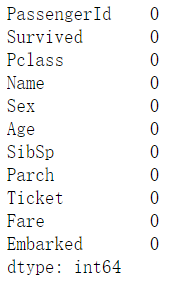2.2 重复值观察与处理

2.2.1 任务一：请查看数据中的重复值

for i in range(df.columns.shape):
print(df.columns[i], 'duplicate', df.duplicated(subset=df.columns[i]).sum())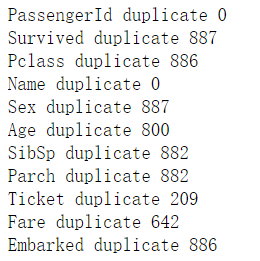2.2.2 任务二：对重复值进行处理

df.drop_duplicates(inplace=True)

2.2.3 任务三：将前面清洗的数据保存为csv格式

df.to_csv("test_clear.csv")

2.3 特征观察与处理

我们对特征进行一下观察，可以把特征大概分为两大类：
数值型特征：Survived ，Pclass， Age ，SibSp， Parch， Fare，其中Survived， Pclass为离散型数值特征，Age，SibSp， Parch， Fare为连续型数值特征
文本型特征：Name， Sex， Cabin，Embarked， Ticket，其中Sex， Cabin， Embarked， Ticket为类别型文本特征，数值型特征一般可以直接用于模型的训练，但有时候为了模型的稳定性及鲁棒性会对连续变量进行离散化。文本型特征往往需要转换成数值型特征才能用于建模分析。

2.3.1 任务一：对年龄进行分箱（离散化）处理

(1) 分箱操作是什么？
可以将连续数值转换成为离散区间。

(2) 将连续变量Age平均分箱成5个年龄段，并分别用类别变量12345表示

df.loc[:, 'AgeBand'] = pd.cut(df.loc[:, 'Age'], bins=5, labels=[1,2,3,4,5])

(3) 将连续变量Age划分为[0,5) [5,15) [15,30) [30,50) [50,80)五个年龄段，并分别用类别变量12345表示

bins = [0, 5, 15, 30, 50, 80]

df.loc[:, 'AgeBand'] = pd.cut(df.loc[:, 'Age'], bins = bins, right=False, labels=[1,2,3,4,5])

(4) 将连续变量Age按10% 30% 50% 70% 90%五个年龄段，并用分类变量12345表示

q = [0, 0.1, 0.3, 0.5, 0.7, 0.9]
df.loc[:, 'AgeBand'] = pd.qcut(df.loc[:, 'Age'], q = q, labels=[1,2,3,4,5])

(5) 将上面的获得的数据分别进行保存，保存为csv格式

2.3.2 任务二：对文本变量进行转换

(1) 查看文本变量名及种类

#方法一: value_counts
df.loc[:, 'Sex'].value_counts()
#方法二: unique
df.loc[:, 'Sex'].unique()

(2) 将文本变量Sex， Cabin ，Embarked用数值变量12345表示

#方法一: replace
df.loc[:, 'Sex_num'] = df.loc[:, 'Sex'].replace(['male', 'female'], [0, 1])

#方法二: map
df.loc[:, 'Sex_num'] = df.loc[:, 'Sex'].map({'male':0, 'female':1})

#方法三: 使用sklearn.preprocessing的LabelEncoder
from sklearn.preprocessing import LabelEncoder
lbl = LabelEncoder()
lbl.fit_transform(df.loc[:, 'Sex'])

(3) 将文本变量Sex， Cabin， Embarked用one-hot编码表示

#方法一: 使用sklearn.preprocessing的OneHotEncoder
from sklearn.preprocessing import OneHotEncoder
ohenc = OneHotEncoder()
df.loc[:, ['male','female']] = ohenc.fit_transform(np.array(df.loc[:, 'Sex_num']).reshape(-1,1)).toarray()

#方法二: 使用get_dummies
pd.get_dummies(df.loc[:, 'Sex'])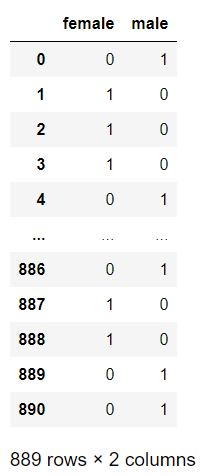2.3.3 任务三：从纯文本Name特征里提取出Titles的特征(所谓的Titles就是Mr,Miss,Mrs等)

df.Name.str.extract('([a-zA-z]+\.)')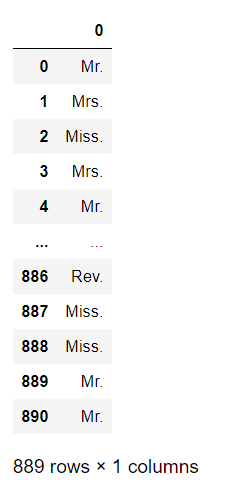df.Name.str.extract('([a-zA-z]+\.)').value_counts()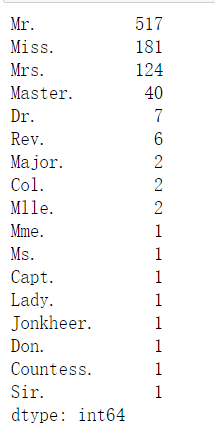展开全文python 数据分析
• 泰坦尼克数据集预测分析Imagine your group of friends have decided to spend the vacations by travelling to an amazing destination. And you have been given the responsibility to find one. Interesting? ...

泰坦尼克数据集预测分析

Imagine your group of friends have decided to spend the vacations by travelling to an amazing destination. And you have been given the responsibility to find one. Interesting? However interesting it may seem, choosing a single location for which everyone agrees is still a hectic task. There are various factors that need to be considered while choosing the location. The cost of the travel journey to the location should fit everyone's pockets. There should be proper accommodation options. The remoteness of the location, activities available, the best time to visit the locations and so on.

想象一下，您的一群朋友决定通过前往一个奇妙的目的地来度过假期。 并且您有责任找到一个。 有趣？ 无论看起来多么有趣，选择每个人都同意的单一位置仍然是一项繁重的任务。 选择位置时，需要考虑多种因素。 前往该地点的旅行费用应适合每个人的腰包。 应该有适当的住宿选择。 位置的偏远性，可用的活动访问位置的最佳时间等。

To get the information about all these factors you have to search on the internet and get information from various sources. After getting all these information, you then have to compare and find a right trade-off between the factors of these locations. The very same activity of gathering, understanding and comparing the data(can be in a visual representation) to help make better decisions is known as Exploratory Data Analysis.

要获取有关所有这些因素的信息，您必须在Internet上搜索并从各种来源获取信息。 在获得所有这些信息之后，您必须比较并在这些位置的因素之间找到正确的权衡。 收集理解比较数据(可以以视觉方式表示)以帮助做出更好的决策的相同活动被称为探索性数据分析

In this article we’ll be Exploring the data of the legendary — ‘Titanic’. We will be using ‘Titanic: Machine Learning from Disaster’ data-set on Kaggle to dive deep into it. The main objective, however, is to predict the survival of the passengers based on the attributes given, here, we’ll be exploring the data-set to find the Hidden Story which was covered along with Sinking of the Titanic. We’ll be unraveling some amazing mysteries behind the sinking of Titanic which you hardly might have heard of. So, get in your detective hat and magnifying glass, ‘coz we’ll be exploring History’s one of the most interesting data till date!

在本文中，我们将探索传说中的“泰坦尼克号”数据。 我们将使用Kaggle上的“ 泰坦尼克号：从灾难中学习机器 ”数据集来深入研究它。 但是，主要目的是根据给定的属性预测乘客的生存，在这里，我们将探索数据集，以查找《泰坦尼克号沉没》所涵盖的隐藏故事 。 在泰坦尼克号沉没的背后，我们将揭开一些令人难以置信的神秘面纱 ，您可能几乎没有听说过。 因此，戴上您的侦探帽和放大镜，因为到目前为止，我们将探索历史上最有趣的数据之一！

I have also provided the link to the Google Colab Notebook having all these code.

To import the data from Kaggle into Google Colab follow these steps—

要将数据从Kaggle导入Go​​ogle Colab，请按照以下步骤操作：

转到您的帐户，单击“创建新的API令牌”，它将在您的计算机上下载kaggle.json文件。
2. Go to your Google Colab project file and run the following commands:

创建名为kaggle的目录，并将kaggle.json文件复制到其中。(Make directory named kaggle and copy kaggle.json file into it.)

Make sure the Drive is Mounted and appropriate folders are created . Here in my case folders Projects>datasets were created where we’ll be moving the downloaded data-set to avoid importing from kaggle repeatedly.

确保已安装驱动器并创建了适当的文件夹。 在我的案例中，这里创建了Projects> datasets文件夹，我们将在其中移动下载的数据集，以避免重复从kaggle导入。

Congratulations, you’ve successfully imported the data-set from Kaggle and stored it into your Google Drive. Next time whenever we need the data-set we can do so by simply copying the path of the file and loading it.

Now, let’s start by importing the required libraries

现在，让我们开始导入所需的库

Before jumping into EDA let’s first load the data-set and have quick glance on it. Below are the libraries that we may require.

在进入EDA之前，我们首先加载数据集并快速浏览一下。 以下是我们可能需要的库。

The data can be loaded in the format of Pandas’ Dataframe as follows and ‘data.shape’ print the dimensions of the data where 891 represents the number of records and 12 represents the number of attributes. Now the path to ‘train.csv’ may vary as per your file’s location. You may directly copy and paste the path to the file in ‘Files’ section right below the ‘Table of Contents’ section (As on year 2020).

数据可以如下所示以Pandas的Dataframe格式加载，“ data.shape”打印数据的尺寸，其中891代表记录数，12代表属性数。 现在，根据您文件的位置，“ train.csv”的路径可能会有所不同。 您可以直接将路径复制并粘贴到“目录”部分下方的“文件”部分中(截至2020年)。

Output:

输出：

Viewing an entire data-set at once can be confusing. So, let’s view some sample of the data. ‘data.head()’ gives the ‘starting 5’ and ‘data.tail()’ gives the bottom 5 records/rows of the dataframe based on the index of the row.

Output:

输出：

Now, let’s print the columns of the dataframe.

现在，让我们打印数据框的列。

Output:

输出：

‘data.info()’ gives information about each attribute and the count of non-null/ non-missing values in each attribute and its datatype. As you can see in the output, the attributes, ‘Age’, ‘Cabin’ and ‘Embarked’ have some missing values present in them.(The processing of these missing values will be done in later modules.)

“ data.info()”提供有关每个属性以及每个属性及其数据类型中非空/非缺失值的计数的信息。 从输出中可以看到，属性'Age'，'Cabin'和'Embarked'中存在一些缺失值(这些缺失值的处理将在以后的模块中完成)。

Output:

输出：

If you have numerical data in the data-set, ‘data.describe()’ can be used to get count, standard deviation, mean and five number summary i.e minimum, 25%(Q1), 50%(median), 75%(Q3) and maximum of each attribute.

如果数据集中有数字数据，则可以使用“ data.describe()”来获取计数，标准偏差，均值和五个数字摘要，即最小值，25％(Q1)，50％(中位数)，75％ (Q3)和每个属性的最大值。

Output:

输出：

了解数据 (Understanding the data)

Okay, so we’ve seen the samples of the data. But what does each of the attributes denote. The description of the attributes are provided in the Kaggle itself. But I’ll try to explain it here to get a better gist of it.

好的，我们已经看到了数据样本。 但是每个属性代表什么。 属性的说明在Kaggle本身中提供。 但是，我将在这里尝试解释它，以便更好地理解它。

There are a total of 891 instances, each consisting of 12 attributes. So here’s a brief information about what the data consist of-

共有891个实例，每个实例包含12个属性。 因此，这是有关数据组成的简要信息-

1. Passenger Id: A unique id given for each passenger in the data-set.

乘客ID ：为数据集中的每个乘客提供的唯一ID。

2. Survived: It denotes whether the passenger survived or not.

2.幸存 ：表示乘客是否幸存。

Here,

这里，

• 0 = Not Survived

0 =未幸存
• 1 = Survived

1 =幸存

3. Pclass: Pclass represents the Ticket class which is also considered as proxy for socio-economic status (SES)

3. Pclass ：Pclass代表票证类，也被视为社会经济地位(SES)的代理

Here,

这里，

• 1 = Upper Class

1 =上层阶级
• 2 = Middle Class

2 =中产阶级
• 3 = Lower Class

3 =下层阶级

4. Name: Name of the Passenger

4.姓名 ：旅客姓名

5. Sex: Denotes the Sex/Gender of the passenger i.e ‘male’ or ‘female’.

5.性别 ：表示乘客的性别/性别，即“男性”或“女性”。

6. Age: Denotes the age of the passenger

6.年龄 ：表示乘客的年龄

Note: If the passenger’s a baby then it’s age is represented in fraction. e.g. 0.33. If the age is estimated, is it in the form of xx.5. e.g. 18.5

注意：如果乘客是婴儿，则年龄以分数表示。 例如0.33。 如果估计年龄，则采用xx.5的形式。 例如18.5

7. SibSp: It represents no. of siblings / spouses aboard the Titanic

7. SibSp ：它代表否。 泰坦尼克号上的兄弟姐妹/配偶

The data-set defines family relations in this way…

数据集以这种方式定义了家庭关系…

• Sibling = brother, sister, stepbrother, stepsister

兄弟姐妹=兄弟，姐妹，继兄弟，继父
• Spouse = husband, wife (mistresses and fiances were ignored)

配偶=丈夫，妻子(情妇和未婚夫被忽略)

8. Parch: It represents no. of parents / children aboard the Titanic

8. Parch ：代表否。 泰坦尼克号上的父母/子女总数

The dataset defines family relations in this way…

数据集以这种方式定义家庭关系…

• Parent = mother, father

父母=母亲，父亲
• Child = daughter, son, stepdaughter, stepson

孩子=女儿，儿子，继女，继子
• Some children travelled only with a nanny, therefore parch=0 for them.

一些孩子只带一个保姆旅行，因此他们的parch = 0。

9. Ticket: It represents the ticket number of the passenger

9.机票 ：代表乘客的机票号码

10. Fare: It represents Passenger fare.

10.票价 ：代表旅客票价。

11. Cabin: It represents the Cabin No.

11.机舱 ：代表机舱号。

12. Embarked: It represents the Port of Embarkation

12.登船 ：代表登船港

Here,

这里，

• C = Cherbourg

C =瑟堡
• Q = Queenstown

Q =皇后镇
• S = Southampton

S =南安普敦

Okay, so now that we have understood the data, let’s hop on to understand the relation between each of the attributes and understand what factors played a major role in the Survival of a Passenger and to also predict if you were in the Titanic, would you have survived or not? Click on the Link to the next story to find out!

好的，现在我们已经了解了数据，让我们开始了解每个属性之间的关系，并了解哪些因素在旅客的生存中起着重要作用，并预测您是否在泰坦尼克号上，幸存了没有？ 单击链接到下一个故事以查找！

链接到笔记本： 单击此处

链接到博客的第2部分： 单击此处

泰坦尼克数据集预测分析

展开全文数据分析 机器学习 python 数据挖掘 大数据
• 经典又兼具备趣味性的案例泰坦尼克号问题源码。大家都熟悉的『Jack and Rose』的故事，豪华游艇倒了，大家都惊恐逃生，可是救生艇的数量有限，无法人人都有，副船长发话了『lady and kid first！』，所以是否获救...泰坦尼克 生存预测
• 基于机器学习的泰坦尼克数据集数据分析 数据集以及ipynb文件放在了我的GitHub上面：欢迎自取 泰坦尼克数据集 任务：预测泰坦尼克乘客生存概率 数据集：train.csv、test.csv 训练集应用于构建您的机器学习模型。 ...

基于机器学习的泰坦尼克数据集数据分析

数据集以及ipynb文件放在了我的GitHub上面：欢迎自取
Titanic - Machine Learning from Disaster

如果本文对你有帮助，欢迎点赞、订阅以及star我的项目。
你的支持是我创作的最大动力！

泰坦尼克数据集

任务：预测泰坦尼克乘客生存概率

数据集：train.csv、test.csv

1. 训练集应用于构建您的机器学习模型。 对于训练集，我们为每位乘客提供结果（也称为“特征”）。 您的模型将基于乘客的性别和阶级等“特征”。 您还可以使用特征工程来创建新特征

2. 应该使用测试集来查看您的模型在看不见的数据上的表现如何。 对于测试集，我们不提供每位乘客的基本情况。 预测这些结果是您的工作。 对于测试集中的每位乘客，请使用您训练的模型来预测他们是否在泰坦尼克号沉没中幸存了下来

#   数据分析
import pandas as pd
import numpy as np
import random as rnd

#   可视化
import seaborn as sns
import matplotlib.pyplot as plt
%matplotlib inline

#   机器学习
from sklearn.linear_model import LogisticRegression
from sklearn.svm import SVC, LinearSVC
from sklearn.ensemble import RandomForestClassifier
from sklearn.neighbors import KNeighborsClassifier
from sklearn.naive_bayes import GaussianNB
from sklearn.linear_model import Perceptron
from sklearn.linear_model import SGDClassifier
from sklearn.tree import DecisionTreeClassifier

combine = [train_df, test_df]

print(train_df.columns.values)

train_df.tail()
train_df.info()
test_df.info()
train_df.describe()

#   Pclass
train_df[['Pclass', 'Survived']].groupby(['Pclass'], as_index=False).mean().sort_values(by='Survived', ascending=False)

#   Sex
train_df[['Sex', 'Survived']].groupby(['Sex'], as_index=False).mean().sort_values(by='Survived', ascending=False)

#  Parch
train_df[['Parch', 'Survived']].groupby(['Parch'], as_index=False).mean().sort_values(by='Survived', ascending=False)

#  可视化数据
g = sns.FacetGrid(train_df, col='Survived')
g.map(plt.hist, 'Age', bins=20)

g = sns.FacetGrid(train_df, col='Survived')
g.map(plt.hist, 'Sex', bins=20)

grid = sns.FacetGrid(train_df, col='Survived', row='Pclass', size=2.2, aspect=1.6)
grid.map(plt.hist, 'Age', alpha=.5, bins=20)

grid = sns.FacetGrid(train_df, row='Embarked', size=2.2, aspect=1.6)
grid.map(sns.pointplot, 'Pclass', 'Survived', 'Sex', palette='deep')

grid = sns.FacetGrid(train_df, row='Embarked', size=2.2, aspect=1.6)
grid.map(sns.barplot, 'Sex', 'Fare', alpha=.5, ci=None)

print("Before", train_df.shape, test_df.shape, combine.shape, combine.shape)

train_df = train_df.drop(['Ticket', 'Cabin'], axis=1)
test_df = test_df.drop(['Ticket', 'Cabin'], axis=1)
combine = [train_df, test_df]

"After", train_df.shape, test_df.shape, combine.shape, combine.shape

for dataset in combine:
dataset['Title'] = dataset.Name.str.extract(' ([A-Za-z]+)\.', expand=False)

pd.crosstab(train_df['Title'], train_df['Sex'])

for dataset in combine:
'Don', 'Dr', 'Major', 'Rev', 'Sir', 'Jonkheer', 'Dona'], 'Rare')
dataset['Title'] = dataset['Title'].replace('Mlle', 'Miss')
dataset['Title'] = dataset['Title'].replace('Ms', 'Miss')
dataset['Title'] = dataset['Title'].replace('Mme', 'Mrs')

train_df[['Title', 'Survived']].groupby(['Title'], as_index=False).mean()

title_mapping = {"Mr": 1, "Miss": 2, "Mrs": 3, "Master": 4, "Rare": 5}
for dataset in combine:
dataset['Title'] = dataset['Title'].map(title_mapping)
dataset['Title'] = dataset['Title'].fillna(0)

train_df = train_df.drop(['Name', 'PassengerId'], axis=1)
test_df = test_df.drop(['Name'], axis=1)
combine = [train_df, test_df]
train_df.shape, test_df.shape

for dataset in combine:
dataset['Sex'] = dataset['Sex'].map({'female':1, 'male':0}).astype(int)

grid = sns.FacetGrid(train_df, row='Pclass', col='Sex', size=2.2, aspect=1.6)
grid.map(plt.hist, 'Age', alpha=.5, bins=20)

guess_ages = np.zeros((2,3))
guess_ages

for dataset in combine:
for i in range(0, 2):
for j in range(0, 3):
guess_df = dataset[(dataset['Sex'] == i) & \
(dataset['Pclass'] == j+1)]['Age'].dropna()

# age_mean = guess_df.mean()
# age_std = guess_df.std()
# age_guess = rnd.uniform(age_mean - age_std, age_mean + age_std)

age_guess = guess_df.median()

# Convert random age float to nearest .5 age
guess_ages[i,j] = int( age_guess/0.5 + 0.5 ) * 0.5

for i in range(0, 2):
for j in range(0, 3):
dataset.loc[ (dataset.Age.isnull()) & (dataset.Sex == i) & (dataset.Pclass == j+1),\
'Age'] = guess_ages[i,j]

dataset['Age'] = dataset['Age'].astype(int)

train_df['AgeBand'] = pd.cut(train_df['Age'], 5)
train_df[['AgeBand', 'Survived']].groupby('AgeBand', as_index=False).mean().sort_values(by='AgeBand', ascending=True)

for dataset in combine:
dataset.loc[ dataset['Age'] <= 16, 'Age'] = 0
dataset.loc[(dataset['Age'] > 16) & (dataset['Age'] <= 32), 'Age'] = 1
dataset.loc[(dataset['Age'] > 32) & (dataset['Age'] <= 48), 'Age'] = 2
dataset.loc[(dataset['Age'] > 48) & (dataset['Age'] <= 64), 'Age'] = 3
dataset.loc[ dataset['Age'] > 64, 'Age']

train_df = train_df.drop(['AgeBand'], axis=1)
combine = [train_df, test_df]

for dataset in combine:
dataset['FamilySize'] = dataset['SibSp'] + dataset['Parch'] + 1

train_df[['FamilySize', 'Survived']].groupby(['FamilySize'], as_index=False).mean().sort_values(by='Survived', ascending=False)

for dataset in combine:
dataset['IsAlone'] = 0
dataset.loc[dataset['FamilySize'] == 1, 'IsAlone'] = 1

train_df[['IsAlone', 'Survived']].groupby(['IsAlone'], as_index=False).mean()

train_df = train_df.drop(['Parch', 'SibSp', 'FamilySize'], axis=1)
test_df = test_df.drop(['Parch', 'SibSp', 'FamilySize'], axis=1)
combine = [train_df, test_df]

for dataset in combine:
dataset['Age*Class'] = dataset.Age * dataset.Pclass

freq_port = train_df.Embarked.dropna().mode()
freq_port

for dataset in combine:
dataset['Embarked'] = dataset['Embarked'].fillna(freq_port)

train_df[['Embarked', 'Survived']].groupby(['Embarked'], as_index=False).mean().sort_values(by='Survived', ascending=False)

for dataset in combine:
dataset['Embarked'] = dataset['Embarked'].map( {'S': 0, 'C': 1, 'Q': 2} ).astype(int)

test_df['Fare'].fillna(test_df['Fare'].dropna().median(), inplace=True)

train_df['FareBand'] = pd.qcut(train_df['Fare'], 4)
train_df[['FareBand', 'Survived']].groupby(['FareBand'], as_index=False).mean().sort_values(by='FareBand', ascending=True)

for dataset in combine:
dataset.loc[ dataset['Fare'] <= 7.91, 'Fare'] = 0
dataset.loc[(dataset['Fare'] > 7.91) & (dataset['Fare'] <= 14.454), 'Fare'] = 1
dataset.loc[(dataset['Fare'] > 14.454) & (dataset['Fare'] <= 31), 'Fare']   = 2
dataset.loc[ dataset['Fare'] > 31, 'Fare'] = 3
dataset['Fare'] = dataset['Fare'].astype(int)

train_df = train_df.drop(['FareBand'], axis=1)
combine = [train_df, test_df]

"""
建模，预测，求解
现在，我们准备训练模型并预测所需的解决方案。 有60多种预测建模算法可供选择。 我们必须了解问题的类型和解决方案的要求，以缩小到可以评估的少数几个模型。 我们的问题是分类和回归问题。 我们要确定输出（是否存活）与其他变量或特征（性别，年龄，港口...）之间的关系。 当我们使用给定的数据集训练模型时，我们也将机器学习称为“监督学习”。 通过这两个标准-监督学习加上分类和回归，我们可以将模型选择范围缩小到几个。 这些包括：
- Logistic Regression
- KNN or k-Nearest Neighbors
- Support Vector Machines
- Naive Bayes classifier
- Decision Tree
- Random Forrest
- Perceptron
- Artificial neural network
- RVM or Relevance Vector Machine
"""

X_train = train_df.drop("Survived", axis=1)
Y_train = train_df["Survived"]
X_test = X_test  = test_df.drop("PassengerId", axis=1).copy()
X_train.shape, Y_train.shape, X_test.shape

# Logistic Regression

logreg = LogisticRegression()
logreg.fit(X_train, Y_train)
Y_pred = logreg.predict(X_test)
acc_log = round(logreg.score(X_train, Y_train) * 100, 2)
acc_log

coeff_df = pd.DataFrame(train_df.columns.delete(0))
coeff_df.columns = ['Feature']
coeff_df["Correlation"] = pd.Series(logreg.coef_)

coeff_df.sort_values(by='Correlation', ascending=False)

# Support Vector Machines

svc = SVC()
svc.fit(X_train, Y_train)
Y_pred = svc.predict(X_test)
acc_svc = round(svc.score(X_train, Y_train) * 100, 2)
acc_svc

# KNN
knn = KNeighborsClassifier(n_neighbors = 3)
knn.fit(X_train, Y_train)
Y_pred = knn.predict(X_test)
acc_knn = round(knn.score(X_train, Y_train) * 100, 2)
acc_knn

# Gaussian Naive Bayes

gaussian = GaussianNB()
gaussian.fit(X_train, Y_train)
Y_pred = gaussian.predict(X_test)
acc_gaussian = round(gaussian.score(X_train, Y_train) * 100, 2)
acc_gaussian

# Linear SVC

linear_svc = LinearSVC()
linear_svc.fit(X_train, Y_train)
Y_pred = linear_svc.predict(X_test)
acc_linear_svc = round(linear_svc.score(X_train, Y_train) * 100, 2)
acc_linear_svc

sgd = SGDClassifier()
sgd.fit(X_train, Y_train)
Y_pred = sgd.predict(X_test)
acc_sgd = round(sgd.score(X_train, Y_train) * 100, 2)
acc_sgd

# Decision Tree

decision_tree = DecisionTreeClassifier()
decision_tree.fit(X_train, Y_train)
Y_pred = decision_tree.predict(X_test)
acc_decision_tree = round(decision_tree.score(X_train, Y_train) * 100, 2)
acc_decision_tree

# Random Forest

random_forest = RandomForestClassifier(n_estimators=100)
random_forest.fit(X_train, Y_train)
Y_pred = random_forest.predict(X_test)
random_forest.score(X_train, Y_train)
acc_random_forest = round(random_forest.score(X_train, Y_train) * 100, 2)
acc_random_forest

models = pd.DataFrame({
'Model': ['Support Vector Machines', 'KNN', 'Logistic Regression',
'Random Forest', 'Naive Bayes', 'Perceptron',
'Decision Tree'],
'Score': [acc_svc, acc_knn, acc_log,
acc_random_forest, acc_gaussian, acc_perceptron,
acc_sgd, acc_linear_svc, acc_decision_tree]})
models.sort_values(by='Score', ascending=False)

submission = pd.DataFrame({
"PassengerId": test_df["PassengerId"],
"Survived": Y_pred
})
# submission.to_csv('../output/submission.csv', index=False)
print(submission)

展开全文算法 机器学习 深度学习 人工智能 数据挖掘
• 泰坦尼克数据集，完善了Fare缺失，保留了Age和Carbin缺失数据挖掘
• 里面包含两个数据集，包含字段信息，PassengerId,Survived,Pclass,Name,Sex,Age,SibSp,Parch,Ticket,Fare,Cabin,Embarked等。
• 泰坦尼克数据集下载 1.1.1 任务一：导入numpy和pandas import numpy as np import pandas as pd 1.1.2 任务二：载入数据 (1) 使用相对路径载入数据 df = pd.read_csv("train.csv")#相对路径 df.head() (2) 使用...

最近一系列的数据分析的内容来源于DataWhale开源

1 第一章：数据载入及初步观察

1.1 载入数据

泰坦尼克号数据集下载

1.1.1 任务一：导入numpy和pandas

import numpy as np
import pandas as pd

1.1.2 任务二：载入数据

(1) 使用相对路径载入数据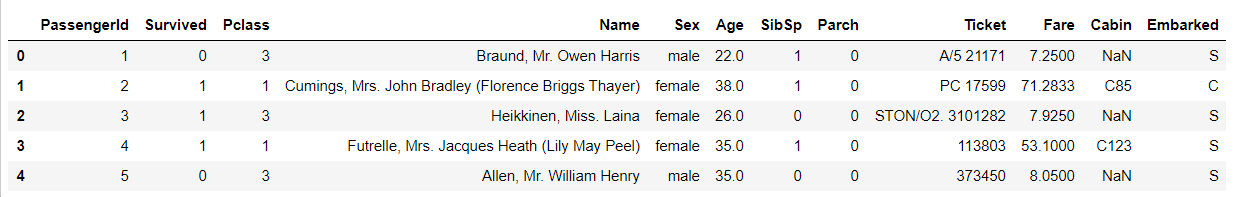(2) 使用绝对路径载入数据

import os
os.getcwd() #查看当前工作目录

1.1.3 任务三：每1000行为一个数据模块，逐块读取

for chunk in chunker:
print(chunk)

chunk的属性为：

1.1.4 任务四：将表头改成中文，索引改为乘客ID [对于某些英文资料，我们可以通过翻译来更直观的熟悉我们的数据]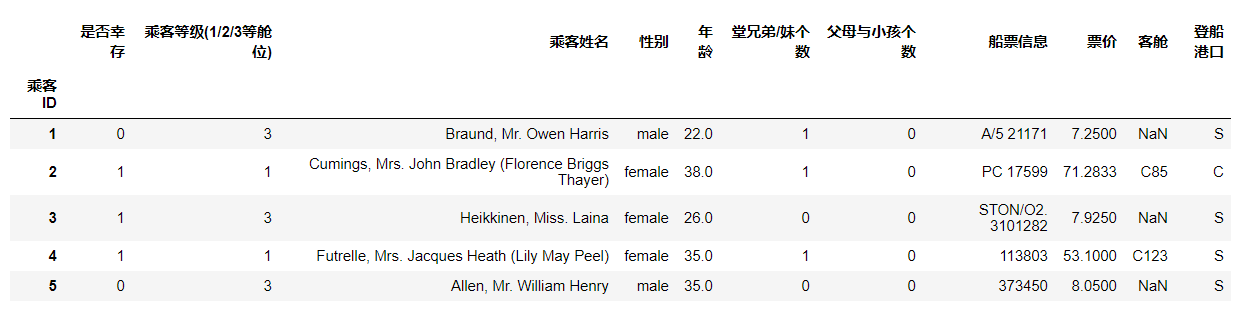1.2 初步观察

1.2.1 任务一：查看数据的基本信息

df.info()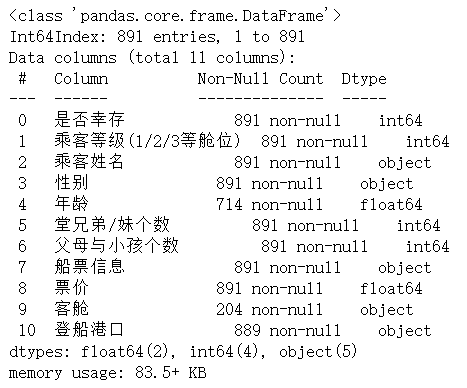1.2.2 任务二：观察表格前10行的数据和后15行的数据

#前10行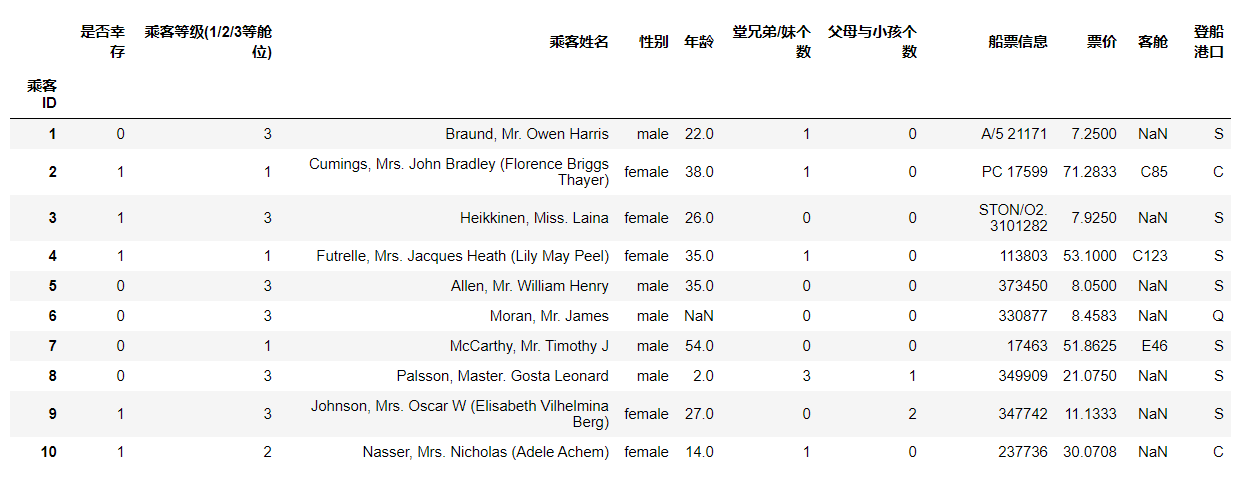#后15行
df.tail(15)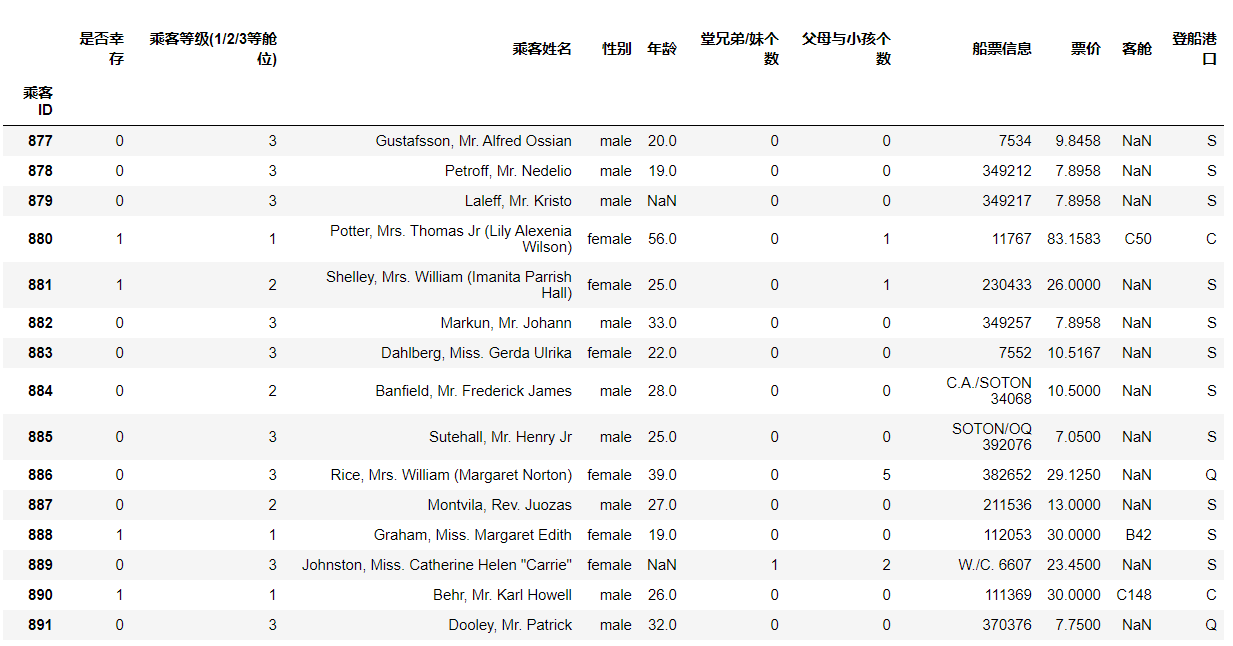1.2.4 任务三：判断数据是否为空，为空的地方返回True，其余地方返回False

df.isnull()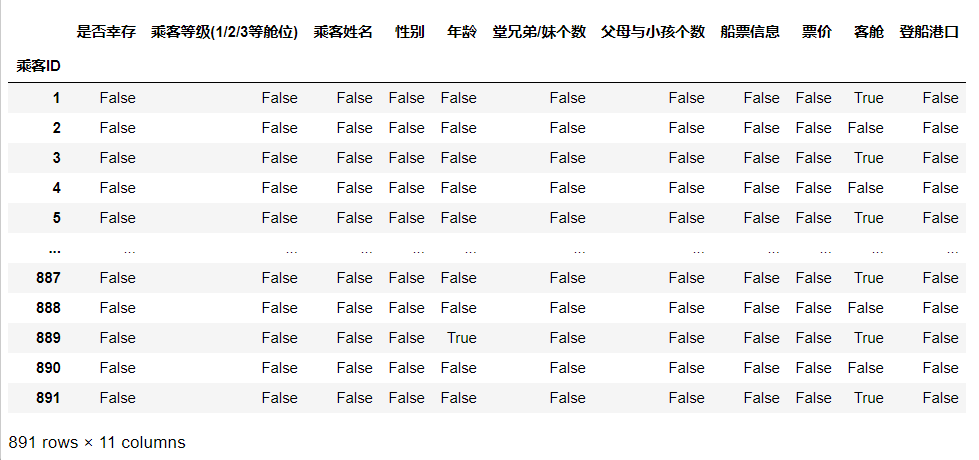1.3 保存数据

1.3.1 任务一：将你加载并做出改变的数据，在工作目录下保存为一个新文件train_chinese.csv

df.to_csv("train_chinese.csv")

【总结】数据的加载以及入门，接下来就要接触数据本身的运算，我们将主要掌握numpy和pandas在工作和项目场景的运用。

1.4 知道你的数据叫什么

1.4.1 任务一：pandas中有两个数据类型DateFrame和Series，通过查找简单了解他们。然后自己写一个关于这两个数据类型的小例子🌰[开放题]

sdata = {'Ohio': 35000, 'Texas': 71000, 'Oregon': 16000, 'Utah': 5000}
example_1 = pd.Series(sdata)
example_1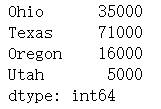'year': [2000, 2001, 2002, 2001, 2002, 2003],'pop': [1.5, 1.7, 3.6, 2.4, 2.9, 3.2]}
example_2 = pd.DataFrame(data)
example_2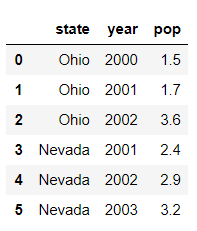Series是一种类似于一维数组的对象。它由一组数据（各种Numpy数据类型）以及一组与之相关的数据标签（即索引）组成。

DataFrame是一个表格型的数据结构，它含有一组有序的列，每列可以是不同的值类型（数值、字符串、布尔型值）。

1.4.3 任务三：查看DataFrame数据的每列的名称

df.columns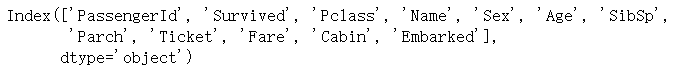1.4.4任务四：查看"Cabin"这列的所有值[有多种方法]

#方法1
df.loc[:,'Cabin']
#方法2
df['Cabin']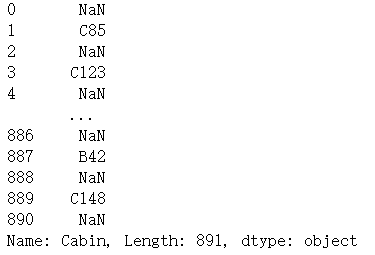1.4.5 任务五：加载文件"test_1.csv"，然后对比"train.csv"，看看有哪些多出的列，然后将多出的列删除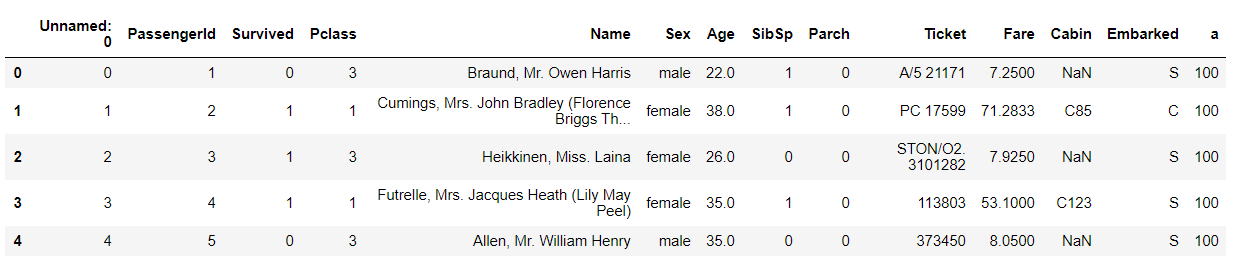df1.columns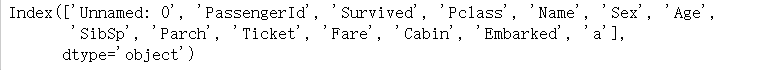same_columns = []
different_columns = []
for i in range(df1.columns.shape):
if df1.columns[i] in df.columns:
same_columns.append(df1.columns[i])
else:
different_columns.append(df1.columns[i])
#删除方法1
for i in range(len(different_columns)):
del df1[different_columns[i]]

#删除方法2
df1.loc[:,different_columns]
df1.drop(different_columns, axis=1, inplace=True)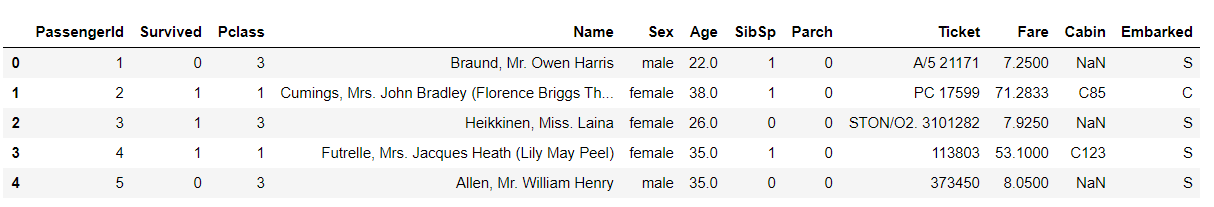1.4.6 任务六： 将[‘PassengerId’,‘Name’,‘Age’,‘Ticket’]这几个列元素隐藏，只观察其他几个列元素

df1.drop(['PassengerId','Name','Age','Ticket'], axis=1)

如果想要完全的删除你的数据结构，使用inplace=True，因为使用inplace就将原数据覆盖了，所以这里没有用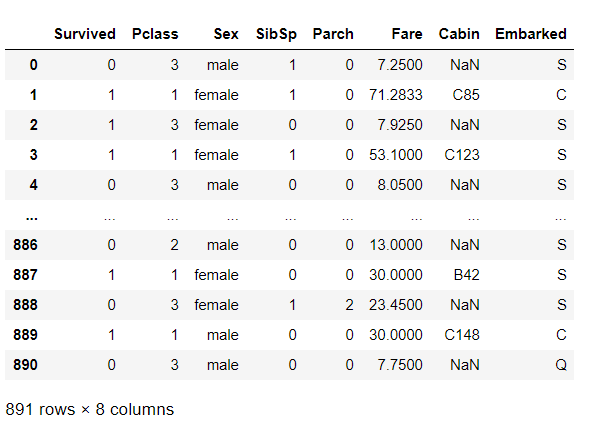1.5 筛选的逻辑

1.5.1 任务一： 我们以"Age"为筛选条件，显示年龄在10岁以下的乘客信息。

df1.loc[df1.loc[:,"Age"] < 10]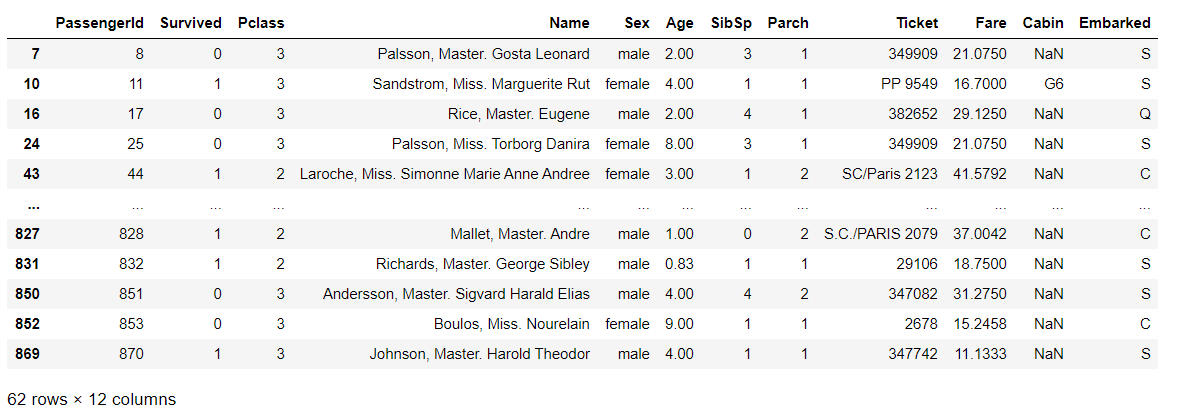1.5.2 任务二： 以"Age"为条件，将年龄在10岁以上和50岁以下的乘客信息显示出来，并将这个数据命名为midage

midage = df1.loc[(df1.loc[:,"Age"] > 10) & (df1.loc[:,"Age"] < 50)]
midage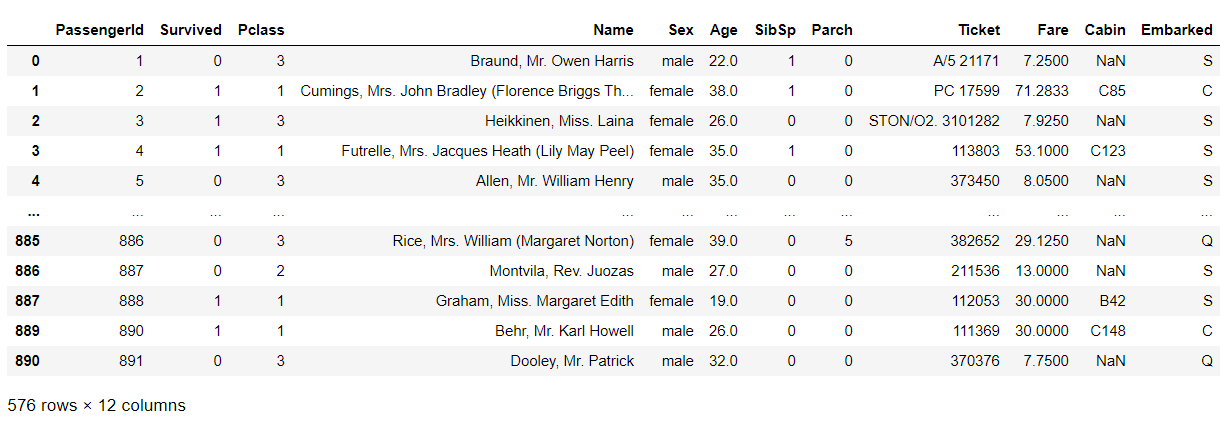1.5.3 任务三：将midage的数据中第100行的"Pclass"和"Sex"的数据显示出来

由于索引乱序，所以需将索引重新排序

midage = midage.reset_index(drop=True)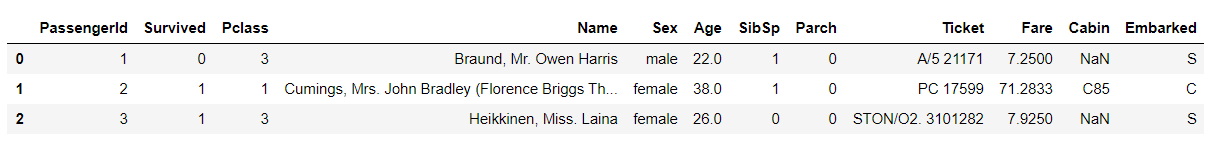midage.loc[, ['Pclass','Sex']]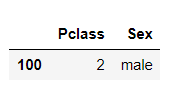1.5.4 任务四：使用loc方法将midage的数据中第100，105，108行的"Pclass"，"Name"和"Sex"的数据显示出来

midage.loc[[100,105,108], ['Pclass','Name','Sex']]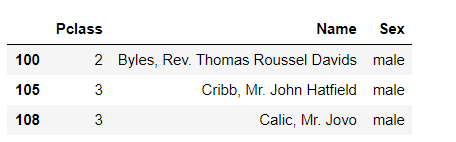1.5.5 任务五：使用iloc方法将midage的数据中第100，105，108行的"Pclass"，"Name"和"Sex"的数据显示出来

midage.iloc[[100,105,108], [2,3,4]]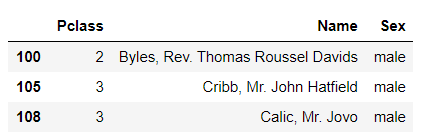【思考】对比iloc和loc的异同

loc使用标签取数据，iloc使用索引取数据

1.6 了解你的数据吗？

1.6.1 任务一：利用Pandas对示例数据进行排序，要求升序

【问题】：大多数时候我们都是想根据列的值来排序,所以将你构建的DataFrame中的数据根据某一列，升序排列

#回答代码
frame = pd.DataFrame(np.arange(8).reshape(2,4)
,index=[2,1]
,columns=['d', 'a', 'b', 'c'])
frame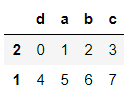frame.sort_index(axis=1)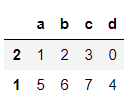【总结】下面将不同的排序方式做一个总结

1.让行索引升序排序

frame.sort_index(axis=0)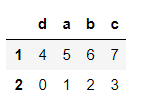2.让列索引升序排序

frame.sort_index(axis=1)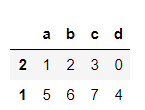3.让列索引降序排序

frame.sort_index(axis=1, ascending=False)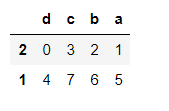4.让任选两列数据同时降序排序

frame.sort_values(by=['a', 'd'], ascending=False)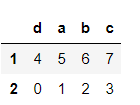1.6.2 任务二：对泰坦尼克号数据（trian.csv）按票价和年龄两列进行综合排序（降序排列），从这个数据中你可以分析出什么？

在开始我们已经导入了train_chinese.csv数据，而且前面我们也学习了导入数据过程，根据上面学习，我们直接对目标列进行排序即可

【思考】排序后，如果我们仅仅关注年龄和票价两列。根据常识我知道发现票价越高的应该客舱越好，所以我们会明显看出，票价前20的乘客中存活的有14人，这是相当高的一个比例，那么我们后面是不是可以进一步分析一下票价和存活之间的关系，年龄和存活之间的关系呢？当你开始发现数据之间的关系了，数据分析就开始了。

多做几个数据的排序

从以上排序看出，仓位等级越好存活率更高，还有女性的存活率更高。

1.6.3 任务三：利用Pandas进行算术计算，计算两个DataFrame数据相加结果

具体请看《利用Python进行数据分析》第五章 算术运算与数据对齐 部分

自己构建两个都为数字的DataFrame数据

“”"
我们举了一个例子：
frame1_a = pd.DataFrame(np.arange(9.).reshape(3, 3),
columns=[‘a’, ‘b’, ‘c’],
index=[‘one’, ‘two’, ‘three’])
frame1_b = pd.DataFrame(np.arange(12.).reshape(4, 3),
columns=[‘a’, ‘e’, ‘c’],
index=[‘first’, ‘one’, ‘two’, ‘second’])
frame1_a
“”"

frame1_a = pd.DataFrame(np.arange(9.).reshape(3, 3),
columns=['a', 'b', 'c'],
index=['one', 'two', 'three'])
frame1_a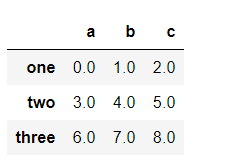frame1_b = pd.DataFrame(np.arange(12.).reshape(4, 3),
columns=['a', 'e', 'c'],
index=['first', 'one', 'two', 'second'])
frame1_b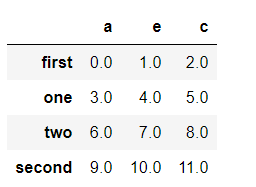将frame_a和frame_b进行相加

frame1_a + frame1_b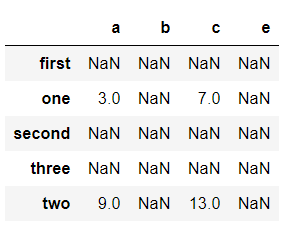【提醒】两个DataFrame相加后，会返回一个新的DataFrame，对应的行和列的值会相加，没有对应的会变成空值NaN。

当然，DataFrame还有很多算术运算，如减法，除法等，有兴趣的同学可以看《利用Python进行数据分析》第五章 算术运算与数据对齐 部分，多在网络上查找相关学习资料。

1.6.4 任务四：通过泰坦尼克号数据如何计算出在船上最大的家族有多少人？

'''
还是用之前导入的chinese_train.csv如果我们想看看在船上，最大的家族有多少人（‘兄弟姐妹个数’+‘父母子女个数’），我们该怎么做呢？
'''
df.loc[:,['兄弟姐妹个数','父母子女个数']]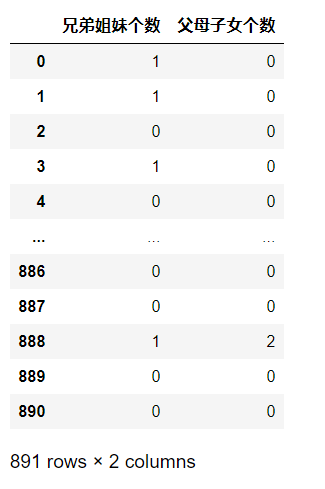max(df['兄弟姐妹个数'] + df['父母子女个数'])

1.6.5 任务五：学会使用Pandas describe()函数查看数据基本统计信息

(1) 关键知识点示例做一遍（简单数据）
具体请看《利用Python进行数据分析》第五章 汇总和计算描述统计 部分

自己构建一个有数字有空值的DataFrame数据

“”"
我们举了一个例子：
frame2 = pd.DataFrame([[1.4, np.nan],
[7.1, -4.5],
[np.nan, np.nan],
[0.75, -1.3]
], index=[‘a’, ‘b’, ‘c’, ‘d’], columns=[‘one’, ‘two’])
frame2

“”"

frame2 = pd.DataFrame([[1.4, np.nan],
[7.1, -4.5],
[np.nan, np.nan],
[0.75, -1.3]
], index=['a', 'b', 'c', 'd'], columns=['one', 'two'])
frame2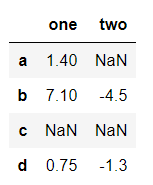调用 describe 函数，观察frame2的数据基本信息

frame2.describe()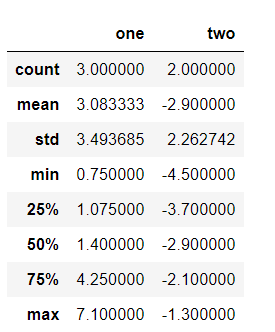1.6.6 任务六：分别看看泰坦尼克号数据集中 票价、父母子女 这列数据的基本统计数据，你能发现什么？

df["票价"].describe()【思考】共有891个票价数据，均值约为:32.20,标准差约为:49.69,最大值约为512.33,75%的票价才约为:31，还未到达平均票价水平，说明票价数据波动较大，且大部分的票价都是较低的，只有小部分票价比较高。

df["父母子女个数"].describe()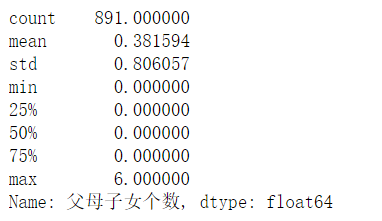【思考】共有891个父母子女个数数据，均值约为:0.38,标准差约为:0.8,最大值约为6,75%的父母子女个数还是0，说明船上大部分人无父母子女，只有极少数人有父母子女。

多做几个组数据的统计，看看你能分析出什么？

df.describe()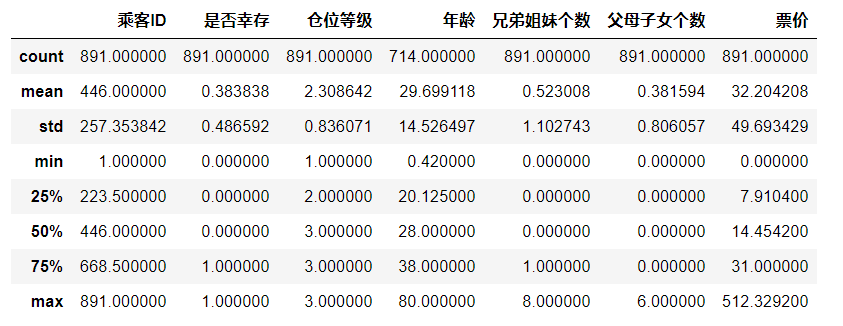【思考】可以看出大部分人的仓位等级为3级，年龄大多为中年人，拥有兄妹的人也只是少数。

【总结】本节中我们通过Pandas的一些内置函数对数据进行了初步统计查看，这个过程最重要的不是大家得掌握这些函数，而是看懂从这些函数出来的数据，构建自己的数据分析思维，这也是第一章最重要的点，希望大家学完第一章能对数据有个基本认识，了解自己在做什么，为什么这么做，后面的章节我们将开始对数据进行清洗，进一步分析。

展开全文• 主要分析有数据接:https://pan.baidu.com/s/1jn88GiOr7uWA8BDQocFXxg 密码: s0e0不同舱位等级中幸存者和遇难者的乘客比例不同性别的幸存比例幸存和遇难...首先做准备（导入库，读入数据集） import matplotlib.pypl
• 泰坦尼克数据中的pclass,sex,age作为决策属性，Survived作类别属性，30%的数据作为测试集，70%作为训练集。用程序得到该数据集的决策树模型，并给出测试数据的准确度。 数据集下载地址： 链接：...决策树 算法 机器学习 python 数据挖掘...